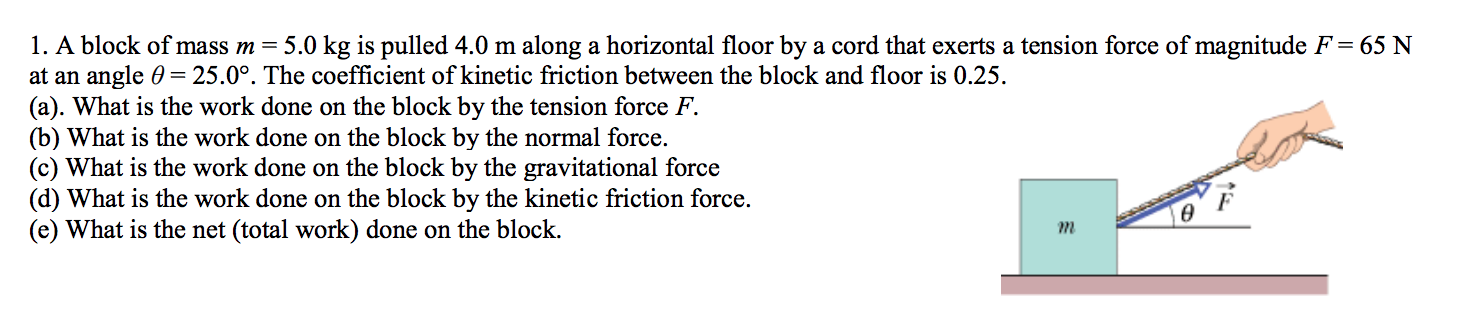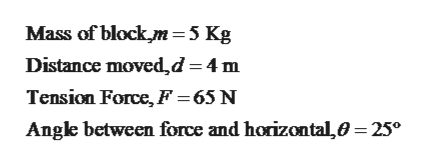# 1. A block of mass m = 5.0 kg is pulled 4.0 m along a horizontal floor by a cord that exerts a tension force of magnitude F = 65 Nat an angle 0 25.0°. The coefficient of kinetic friction between the block and floor is 0.25(a). What is the work done on the block by the tension force F(b) What is the work done on the block by the normal force.(c) What is the work done on the block by the gravitational force(d) What is the work done on the block by the kinetic friction force(e) What is the net (total work) done on the blockm

Question
5 viewshelp_outlineImage Transcriptionclose1. A block of mass m = 5.0 kg is pulled 4.0 m along a horizontal floor by a cord that exerts a tension force of magnitude F = 65 N at an angle 0 25.0°. The coefficient of kinetic friction between the block and floor is 0.25 (a). What is the work done on the block by the tension force F (b) What is the work done on the block by the normal force. (c) What is the work done on the block by the gravitational force (d) What is the work done on the block by the kinetic friction force (e) What is the net (total work) done on the block m fullscreen
check_circle

Step 1

Given,help_outlineImage TranscriptioncloseMass of block m=5 Kg Distance moved,d =4 m Tension Force, F =65 N Angle between force and horizontal,0 25° fullscreen
Step 2

Forces acting on the block can be shown in free body diagram as follows,

Step 3

Work done by any force (F) acti...

### Want to see the full answer?

See Solution

#### Want to see this answer and more?

Solutions are written by subject experts who are available 24/7. Questions are typically answered within 1 hour.*

See Solution
*Response times may vary by subject and question.
Tagged in

### Work,Power and Energy